## Mirror Images

#### Mirror Images

Direction: In each of the following questions you are given a combination of alphabets and/ or numbers followed by four alternatives (a), (b), (c) and (d). Choose the alternatives which is closely resembles the Mirror image of the given combination.

1. DEGREE
1. .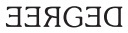2. .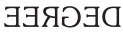3. .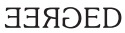4. .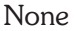1. Answer B

##### Correct Option: B

Answer B

1. 7155 x 7156
1. .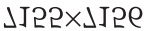2. .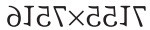3. .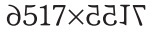4. .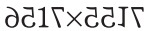1. Answer D

##### Correct Option: D

Answer D

1. SUMMER
1. .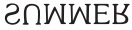2. .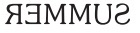3. .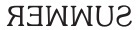4. .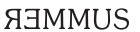1. Answer B

##### Correct Option: B

Answer B

1. GLOBE
1. .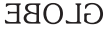2. .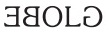3. .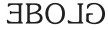4. .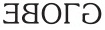1. Answer A

##### Correct Option: A

Answer A

1. BANKING
1. .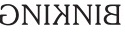2. .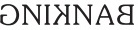3. .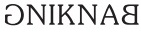4. .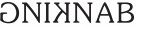1. Answer B

Answer B